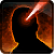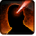# SnipeConditions

Used by

## Related effects, buffs and debuffs

Please click on an effect below to view its details.

• [hidden] [0s] [not displayed]Effect #1

 Slot: Debuff Duration: 0s Tick rate: does not tick # occurrences: 0
• On Apply

Perform the following actions:

• Add effect #2 to TARGET from TARGET
• [hidden] [0s]Effect #2

 Slot: Debuff Duration: 0s Tick rate: does not tick # occurrences: 0 Conditions: Can only by called by other effects
• On Apply

Perform the following actions:

• Play appearance epp . agent . snipe . snipe_fire
• Invoke Projectile
- Determine Hit = (bool) false
- Timed Projectile = (bool) false
- Hit Checked = (bool) false
- Is Special Ability = (bool) true
- Ignore Dual Wield Modifier = (bool) false
- Slot = (int) 2
- Spell Type = (int) 1
- Travel Speed = (float) 10
- Travel Time = (time) 0s
• When projectile completed travel and hit target

Only when the following conditions are met:

• If difficulty is set to 16-man

Perform the following actions:

• Weapon Damage
- Is Special Ability = (bool) true
- Ignore Dual Wield Modifier = (bool) true
- Unknown (609) = (bool) false
- Flurry Blows Min = (int) 1
- Flurry Blows Max = (int) 1
- Level Cap = (int) 0
- Threat Percent = (float) 0
- Standard Health Percent Max = (float) 0.035
- Standard Health Percent Min = (float) 0.035
- Amount Modifier Fixed Max = (float) 0
- Amount Modifier Fixed Min = (float) 0
- Amount Modifier Percent = (float) -1
- Coefficient = (float) 0
- Health Steal Percentage = (float) 0
- Slots = Int(2)
• When projectile completed travel and hit target

Only when the following conditions are met:

• If difficulty is set to 8-man SM

Perform the following actions:

• Weapon Damage
- Is Special Ability = (bool) true
- Ignore Dual Wield Modifier = (bool) true
- Unknown (609) = (bool) false
- Flurry Blows Min = (int) 1
- Flurry Blows Max = (int) 1
- Level Cap = (int) 0
- Threat Percent = (float) 0
- Standard Health Percent Max = (float) 0.07
- Standard Health Percent Min = (float) 0.07
- Amount Modifier Fixed Max = (float) 0
- Amount Modifier Fixed Min = (float) 0
- Amount Modifier Percent = (float) -1
- Coefficient = (float) 0
- Health Steal Percentage = (float) 0
- Slots = Int(2)
• When projectile completed travel and hit target

Only when the following conditions are met:

• If difficulty is set to 8-man HM

Perform the following actions:

• Weapon Damage
- Is Special Ability = (bool) true
- Ignore Dual Wield Modifier = (bool) true
- Unknown (609) = (bool) false
- Flurry Blows Min = (int) 1
- Flurry Blows Max = (int) 1
- Level Cap = (int) 0
- Threat Percent = (float) 0
- Standard Health Percent Max = (float) 0.22
- Standard Health Percent Min = (float) 0.2
- Amount Modifier Fixed Max = (float) 0
- Amount Modifier Fixed Min = (float) 0
- Amount Modifier Percent = (float) -1
- Coefficient = (float) 0
- Health Steal Percentage = (float) 0
- Slots = Int(2)